# Geometric Angles & Transformation Chapter Exam

Exam Instructions:

Choose your answers to the questions and click 'Next' to see the next set of questions. You can skip questions if you would like and come back to them later with the yellow "Go To First Skipped Question" button. When you have completed the practice exam, a green submit button will appear. Click it to see your results. Good luck!

### Page 1

#### Question 1 1. Given the figure below, Angle 3 = 140y and Angle 5 = 80y + 70. Determine the value of y.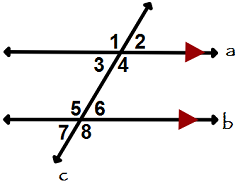#### Question 2 2. What is the relationship between Angle 2 and Angle 7?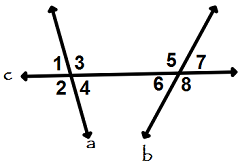#### Question 3 3. If the pictured triangles are congruent, what reason can be given?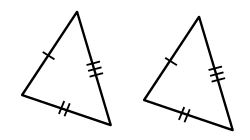#### Question 4 4. If the pictured triangles are congruent, what reason can be given?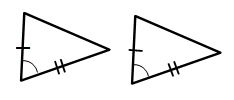### Page 2

#### Question 8 8. If you were to estimate the measure of the angle shown, which of the following would be the best guess?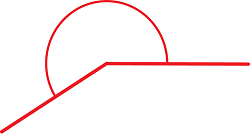#### Question 10 10. Which type of transformation is shown here?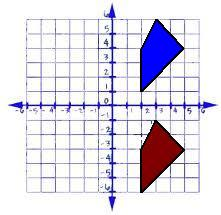### Page 3

#### Question 13 13. Which theorem or postulate can be used to establish congruence with the pictured triangles?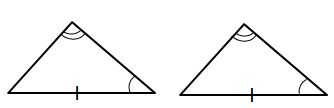### Page 4

#### Question 17 17. Which expression could be used to determine the length of the green arrow?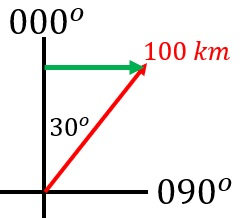#### Question 18 18. Which compass bearing could the purple arrow represent?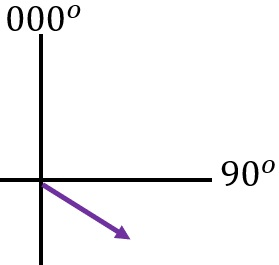### Page 5

#### Question 23 23. Consider the figure below, where Angle 4 = 12m - 4 Angle 5 = 10m + 20 Calculate the measure of Angle 5.#### Question 24 24. If the pictured triangles are congruent, what reason can be given?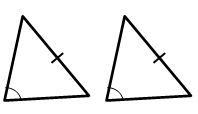### Page 6

#### Question 26 26. The angle shown lands somewhere between _____ and _____, and is about a(n) _____ angle. (Select the most accurate answer)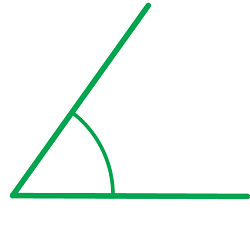#### Question 27 27. Which type of transformation is shown here?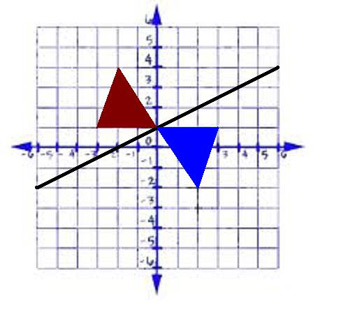#### Question 28 28. Which of the following is a pair of adjacent angles?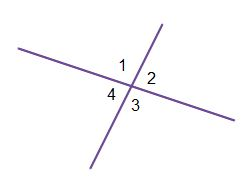#### Question 29 29. Which theorem or postulate can be used to establish congruence with the pictured triangles?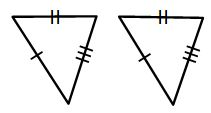#### Geometric Angles & Transformation Chapter Exam Instructions

Choose your answers to the questions and click 'Next' to see the next set of questions. You can skip questions if you would like and come back to them later with the yellow "Go To First Skipped Question" button. When you have completed the practice exam, a green submit button will appear. Click it to see your results. Good luck!

Support